# Fourier expansion electromagnetic field

The electromagnetic (EM) field is of importance as a carrier of solar energy and of electronic signals (radio, TV, etc.). As its name suggests, it consists of two vector fields, the electric field E and the magnetic field B. The Fourier expansion of the electromagnetic field is used in the quantization that leads to photons (light particles of well-defined energy and momentum). Further the Fourier transforms of EM fields play a role in theory of wave propagation through different media and light scattering.

In the absence of charges and electric currents (that is, in "free" space), both E and B can be derived from a third vector field, the vector potential A. Below, the Fourier transform of the three real fields E, B, and A will be introduced. It will be seen that the expansion of the vector potential A yields the expansions of the fields E and B. Further the energy and momentum of the EM field will be expressed in the Fourier components of A.

## Fourier expansion of a vector field

A real scalar function f(x), with 0 ≤ xL, has the following Fourier expansion: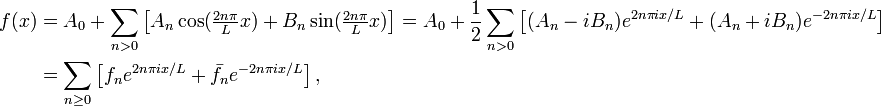\begin{align} f(x) &= A_0 + \sum_{n>0}\left[A_n \cos(\tfrac{2n\pi}{L}x) +B_n \sin(\tfrac{2n\pi}{L}x)\right] =A_0 +\frac{1}{2} \sum_{n>0}\left[ (A_n - iB_n)e^{2n\pi ix/L} + (A_n + iB_n)e^{-2n\pi ix/L}\right]\\ &= \sum_{n\ge 0}\left[ f_n e^{2n\pi ix/L} + \bar{f}_n e^{-2n\pi ix/L}\right], \end{align}

where the bar indicates complex conjugation and the definition of the Fourier components is obvious. Note that the summand consists of a term plus its complex conjugate and hence is real. For a real scalar field f(r) depending on three variables, r ≡ (x, y, z), the Fourier expansion is easily generalized; it is the following:$f(\mathbf{r}, t) = \sum_\mathbf{k} \left( f_k(t) e^{i\mathbf{k}\cdot\mathbf{r}} + \bar{f}_k(t) e^{-i\mathbf{k}\cdot\mathbf{r}} \right),\qquad \mathbf{k} = \frac{2\pi}{L} ( n_x, \; n_y,\; n_z), \quad\hbox{with}\quad n_x,\,n_y,\,n_z = 0,\; 1,\;2,\ldots$

Such an expansion, labeled by a discrete (countable) set of vectors k, is always possible when f satisfies periodic boundary conditions, i.e., f(r + p,t) = f(r,t) for some finite vector p. To impose such boundary conditions, it is common to consider EM waves as if they are in a virtual cubic box of finite volume V = L3. Waves on opposite walls of the box are enforced to have the same value (usually zero). Note that the waves are not restricted to the box: the box is replicated an infinite number of times in x, y, and z direction.

The expansion above is over the first octant of the (kx, ky, kz) lattice. It is often extended to run over all octants, in which case an overcomplete (linearly dependent, not completely orthogonal) basis is used. When furthermore the expansion is applied to the three components of a vector field F(r,t) separately, the result can be written concisely as follows:$\mathbf{F}(\mathbf{r}, t) = \sum_\mathbf{k} \left( \mathbf{f}_k(t) e^{i\mathbf{k}\cdot\mathbf{r}} + \bar{\mathbf{f}}_k(t) e^{-i\mathbf{k}\cdot\mathbf{r}} \right),\qquad \mathbf{k} = \frac{2\pi}{L} ( n_x, \; n_y,\; n_z), \quad\hbox{with}\quad n_x,\,n_y,\,n_z = 0,\pm\; 1,\;\pm2,\ldots$

with the following orthogonality relations,\begin{align} \iiint_V e^{-i\mathbf{k}\cdot\mathbf{r}}e^{ i\mathbf{k'}\cdot\mathbf{r}}\mathrm{d}^3\mathbf{r} &= V \delta_{\mathbf{k},\mathbf{k}'},\quad \iiint_V e^{ i\mathbf{k}\cdot\mathbf{r}}e^{ i\mathbf{k'}\cdot\mathbf{r}}\mathrm{d}^3\mathbf{r} &= V \delta_{\mathbf{k},-\mathbf{k}'},\quad \iiint_V e^{-i\mathbf{k}\cdot\mathbf{r}}e^{-i\mathbf{k'}\cdot\mathbf{r}}\mathrm{d}^3\mathbf{r} &= V \delta_{\mathbf{k},-\mathbf{k}'}. \end{align}

## Vector potential

The magnetic field B satisfies the following Maxwell equation: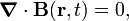$\boldsymbol{\nabla}\cdot \mathbf{B}(\mathbf{r},t) = 0,$

that is, the divergence of B is zero. This equation expresses the fact that magnetic monopoles (charges) do not exist (or, rather, have never been found in nature). A divergence-free field, such as B, is a also referred to as a transverse field. By the Helmholtz decomposition, B can be written as$\mathbf{B}(\mathbf{r}, t) = \boldsymbol{\nabla}\times \mathbf{A}(\mathbf{r}, t),$

in which the vector potential A is introduced though the curl ×A.

The electric field obeys one of the Maxwell equations, in electromagnetic SI units it reads,$\boldsymbol{\nabla}\cdot\mathbf{E}(\mathbf{r},t) = \frac{\rho}{\epsilon_0} = 0$

because it is assumed that charge distributions ρ are zero. The quantity ε0 is the electric constant. Hence, also the electric field E is transverse. Since there are no charges, the electric potential is zero and the electric field follows from A by,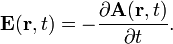$\mathbf{E}(\mathbf{r}, t) = - \frac{\partial \mathbf{A}(\mathbf{r}, t)}{\partial t}.$

The fact that E can be written this way is due to the choice of Coulomb gauge for A:$\boldsymbol{\nabla}\cdot\mathbf{A}(\mathbf{r}, t) = 0.$

By definition, a choice of gauge does not affect any measurable properties (the best known example of a choice of gauge is the fixing of the zero of an electric potential, for instance at infinity). The Coulomb gauge makes A transverse as well, and clearly A is parallel to E. (The time differentiation does not affect direction.) The vector fields A, B, and E are in the same plane, as they are orthogonal to the same longitudinal vector. Hence, the three fields can be written as a linear combination of two orthonormal vectors, ex and ey. It is often convenient to choose complex unit vectors obtained by a unitary transformation,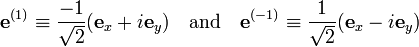$\mathbf{e}^{(1)} \equiv \frac{-1}{\sqrt{2}}(\mathbf{e}_x + i \mathbf{e}_y)\quad\hbox{and}\quad\mathbf{e}^{(-1)} \equiv \frac{1}{\sqrt{2}}(\mathbf{e}_x - i \mathbf{e}_y)$

which are orthonormal,$\mathbf{e}^{(\mu)}\cdot\bar{\mathbf{e}}^{(\mu')} = \delta_{\mu,\mu'}\quad\hbox{with}\quad\mu,\mu'= 1,\, -1,$

and the bar indicating the complex conjugate.

## Expansions

The Fourier expansion of the vector potential reads$\mathbf{A}(\mathbf{r}, t) = \sum_\mathbf{k}\sum_{\mu=-1,1} \left( \mathbf{e}^{(\mu)}(\mathbf{k}) a^{(\mu)}_\mathbf{k}(t) \, e^{i\mathbf{k}\cdot\mathbf{r}} + \bar{\mathbf{e}}^{(\mu)}(\mathbf{k}) \bar{a}^{(\mu)}_\mathbf{k}(t) \, e^{-i\mathbf{k}\cdot\mathbf{r}} \right).$

The vector potential obeys the wave equation,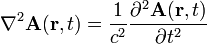$\nabla^2 \mathbf{A}(\mathbf{r},t) = \frac{1}{c^2} \frac{\partial^2 \mathbf{A}(\mathbf{r},t)}{\partial t^2}$

The substitution of the Fourier series of A into the wave equation yields for the individual terms,$-k^2 a^{(\mu)}_\mathbf{k}(t) = \frac{1}{c^2} \frac{\partial^2 a^{(\mu)}_\mathbf{k}(t)}{\partial t^2} \quad \Longrightarrow \quad a^{(\mu)}_\mathbf{k}(t) \propto e^{-i\omega t}\quad\hbox{with}\quad\omega = kc\quad\hbox{and}\quad k \equiv |\mathbf{k}|.$

It is now an easy matter to construct the corresponding Fourier expansions for E and B from the expansion of the vector potential A. The expansion for E follows from differentiation with respect to time,$\mathbf{E}(\mathbf{r}, t) = i\sum_\mathbf{k}\sum_{\mu=-1,1} \omega \left( \mathbf{e}^{(\mu)}(\mathbf{k}) a^{(\mu)}_\mathbf{k}(t) \, e^{i\mathbf{k}\cdot\mathbf{r}} - \bar{\mathbf{e}}^{(\mu)}(\mathbf{k}) \bar{a}^{(\mu)}_\mathbf{k}(t) \, e^{-i\mathbf{k}\cdot\mathbf{r}} \right) .$

The expansion for B follows by taking the curl,$\mathbf{B}(\mathbf{r}, t) = i\sum_\mathbf{k}\sum_{\mu=-1,1} \left( \big[\mathbf{k}\times \mathbf{e}^{(\mu)}(\mathbf{k})\big]\; a^{(\mu)}_\mathbf{k}(t) \, e^{i\mathbf{k}\cdot\mathbf{r}} - \big[\mathbf{k}\times\bar{\mathbf{e}}^{(\mu)}(\mathbf{k})\big]\; \bar{a}^{(\mu)}_\mathbf{k}(t) \, e^{-i\mathbf{k}\cdot\mathbf{r}} \right) .$

## Fourier-expanded energy

The electromagnetic energy density is$\mathcal{E}_\mathrm{Field} = \frac{1}{2} ( \epsilon_0 \mathbf{E}\cdot\mathbf{E}+ \frac{1}{\mu_0}\mathbf{B}\cdot\mathbf{B}),$

where μ0 is the magnetic constant. The total energy (classical Hamiltonian) of a finite volume V is defined by$H = \iiint_V \mathcal{E}_\mathrm{Field}(\mathbf{r},t) \mathrm{d}^3\mathbf{r}.$

Use in the expansion of EE = E2 the following\begin{align} \frac{1}{V}\iiint_V &\left( \mathbf{e}^{(\mu')}(\mathbf{k'}) a^{(\mu')}_\mathbf{k'}(t) \, e^{i\mathbf{k'}\cdot\mathbf{r}} - \bar{\mathbf{e}}^{(\mu')}(\mathbf{k'}) \bar{a}^{(\mu')}_\mathbf{k'}(t) \, e^{-i\mathbf{k'}\cdot\mathbf{r}} \right) \cdot \left( \mathbf{e}^{(\mu)}(\mathbf{k}) a^{(\mu)}_\mathbf{k}(t) \, e^{i\mathbf{k}\cdot\mathbf{r}} - \bar{\mathbf{e}}^{(\mu)}(\mathbf{k}) \bar{a}^{(\mu)}_\mathbf{k}(t) \, e^{-i\mathbf{k}\cdot\mathbf{r}} \right)\mathrm{d}^3\mathbf{r} \\ &= -2\delta_{\mathbf{k'},\mathbf{k}}\delta_{\mu',\mu}\,a^{(\mu)}_\mathbf{k}(t) \bar{a}^{(\mu)}_\mathbf{k}(t)\;+\; \delta_{\mathbf{k'},-\mathbf{k}} \mathbf{e}^{(\mu')}(-\mathbf{k})\cdot\mathbf{e}^{(\mu)}(\mathbf{k}) \left[a^{(\mu')}_\mathbf{k}(t) a^{(\mu)}_\mathbf{-k}(t) + \bar{a}^{(\mu')}_\mathbf{k}(t) \bar{a}^{(\mu)}_\mathbf{-k}(t)\right] \end{align}

The first term (linear in δk',k) will survive and will be added to the same term appearing in the expansion of B2. It may be tempting to consider what the value will be of the following factor,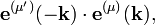$\mathbf{e}^{(\mu')}(-\mathbf{k})\cdot\mathbf{e}^{(\mu)}(\mathbf{k}),$

(appearing in the second term, linear in δk',−k), but this is not needed, because it cancels against the very same expression arising in the expansion of B2.

In the expansion of B2 the following appears as the factor of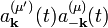$a^{(\mu')}_\mathbf{k}(t) a^{(\mu)}_\mathbf{-k}(t)$  and$\bar{a}^{(\mu')}_\mathbf{k}(t) \bar{a}^{(\mu)}_\mathbf{-k}(t):$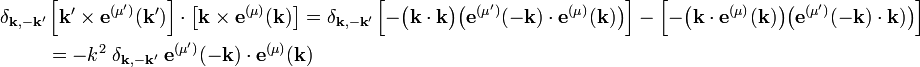\begin{align} \delta_{\mathbf{k},-\mathbf{k}'} & \left[\mathbf{k'}\times\mathbf{e}^{(\mu')}(\mathbf{k'})\right]\cdot \left[\mathbf{k}\times\mathbf{e}^{(\mu)}(\mathbf{k})\right] =\delta_{\mathbf{k},-\mathbf{k}'} \left[ -\big(\mathbf{k}\cdot\mathbf{k}\big) \big(\mathbf{e}^{(\mu')}(-\mathbf{k})\cdot\mathbf{e}^{(\mu)}(\mathbf{k})\big) \right] - \left[ -\big(\mathbf{k}\cdot \mathbf{e}^{(\mu)}(\mathbf{k})\big) \big(\mathbf{e}^{(\mu')}(-\mathbf{k})\cdot\mathbf{k})\big) \right] \\ &= -k^2\;\delta_{\mathbf{k},-\mathbf{k}'}\; \mathbf{e}^{(\mu')}(-\mathbf{k})\cdot\mathbf{e}^{(\mu)}(\mathbf{k}) \end{align}

Recall that:

(i) The expression of the field energy E2 contains a factor ε0 and B2 the factor 1/μ0.

(ii)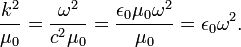$\frac{k^2}{\mu_0} = \frac{\omega^2}{c^2\mu_0} = \frac{\epsilon_0\mu_0 \omega^2}{\mu_0} = \epsilon_0 \omega^2.$

(iii) The Fourier expansion of E contains the factor ω.

This means that all ingredients have been mentioned to arrive at the classical Hamiltonian in terms of Fourier coefficients,$H = 2V\epsilon_0 \sum_\mathbf{k}\sum_{\mu=1,-1} \omega^2 a^{(\mu)}_\mathbf{k}(t)\bar{a}^{(\mu)}_\mathbf{k}(t)\quad\hbox{with}\quad \mathbf{k} = \frac{2\pi}{L} (n_x,\;n_y,\;n_z)\quad \hbox{and}\quad n_x,\; n_y,\; n_z = 0,\;\pm1,\;\pm2,\ldots$

## Fourier-expanded momentum

The electromagnetic momentum, PEM, of EM radiation enclosed by a volume V is proportional to an integral of the Poynting vector S. In SI units: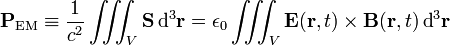\begin{align} \mathbf{P}_\textrm{EM} &\equiv \frac{1}{c^2} \iiint_V \mathbf{S}\, \textrm{d}^3\mathbf{r} = \epsilon_0 \iiint_V \mathbf{E}(\mathbf{r},t)\times \mathbf{B}(\mathbf{r},t)\, \textrm{d}^3\mathbf{r}\\ \end{align}

To show that the expansion terms containing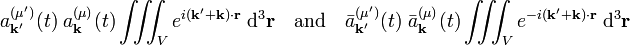$a^{(\mu')}_\mathbf{k'}(t)\;a^{(\mu)}_\mathbf{k}(t) \iiint_V e^{i(\mathbf{k'}+\mathbf{k})\cdot\mathbf{r}} \;\mathrm{d}^3\mathbf{r} \quad\hbox{and}\quad \bar{a}^{(\mu')}_\mathbf{k'}(t)\;\bar{a}^{(\mu)}_\mathbf{k}(t) \iiint_V e^{-i (\mathbf{k'}+\mathbf{k})\cdot\mathbf{r}} \;\mathrm{d}^3\mathbf{r}$

cancel, we write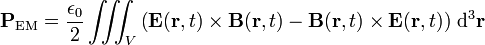$\mathbf{P}_\textrm{EM} = \frac{\epsilon_0}{2} \iiint_V\left( \mathbf{E}(\mathbf{r},t)\times \mathbf{B}(\mathbf{r},t)- \mathbf{B}(\mathbf{r},t)\times \mathbf{E}(\mathbf{r},t)\right)\, \textrm{d}^3\mathbf{r}$

Upon expansion, one finds among other terms,$\delta_{\mathbf{k'},-\mathbf{k}} a^{(\mu')}_\mathbf{k'}(t)\;a^{(\mu)}_\mathbf{k}(t) \Big[ \mathbf{e}^{(\mu')}(\mathbf{k'})\times\left(\mathbf{k}\times \mathbf{e}^{(\mu)}(\mathbf{k}) \right) -\left(\mathbf{k'}\times \mathbf{e}^{(\mu')}(\mathbf{k'}) \right)\times\mathbf{e}^{(\mu)}(\mathbf{k}) \Big]$

Twice the Baccab formula and using that  "under δk',−k"   k and −k' maybe interchanged, and an obvious short-hand notation gives\begin{align} \mathbf{e}'\times(\mathbf{k}\times \mathbf{e}) &= \mathbf{k} (\mathbf{e}'\cdot \mathbf{e}) - \mathbf{e} (\mathbf{e}'\cdot \mathbf{k})= \mathbf{k} (\mathbf{e}'\cdot \mathbf{e}) + \mathbf{e} (\mathbf{e}'\cdot \mathbf{k}') =\mathbf{k} (\mathbf{e}'\cdot \mathbf{e}) \\ -(\mathbf{k}'\times \mathbf{e}')\times\mathbf{e} &= \mathbf{e}\times(\mathbf{k}'\times \mathbf{e}')= \mathbf{k}' (\mathbf{e}\cdot \mathbf{e}') - \mathbf{e}' (\mathbf{e}\cdot \mathbf{k}')= \mathbf{k}' (\mathbf{e}\cdot \mathbf{e}') + \mathbf{e}' (\mathbf{e}\cdot \mathbf{k}) =\mathbf{k}' (\mathbf{e}\cdot \mathbf{e}') . \\ \end{align}

And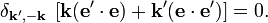$\delta_{\mathbf{k'},-\mathbf{k}}\;\left[ \mathbf{k} (\mathbf{e}'\cdot \mathbf{e}) + \mathbf{k}' (\mathbf{e}\cdot \mathbf{e}') \right] = 0 .$

The other terms in the expansion do not offer any new problems, so that, finally,$\mathbf{P}_\textrm{EM} = 2 V \epsilon_0 \sum_\mathbf{k}\sum_{\mu=1,-1} \omega \mathbf{k} a^{(\mu)}_\mathbf{k}(t)\bar{a}^{(\mu)}_\mathbf{k}(t)\quad\hbox{with}\quad \mathbf{k} = \frac{2\pi}{L} (n_x,\;n_y,\;n_z)\quad \hbox{and}\quad n_x,\; n_y,\; n_z = 0,\;\pm1,\;\pm2,\ldots.$